# 10.8 Vectors  (Page 7/22)

 Page 7 / 22

## Verbal

What are the characteristics of the letters that are commonly used to represent vectors?

lowercase, bold letter, usually $\text{\hspace{0.17em}}u,v,w$

How is a vector more specific than a line segment?

What are $\text{\hspace{0.17em}}i\text{\hspace{0.17em}}$ and $\text{\hspace{0.17em}}j,$ and what do they represent?

They are unit vectors. They are used to represent the horizontal and vertical components of a vector. They each have a magnitude of 1.

What is component form?

When a unit vector is expressed as $⟨a,b⟩,$ which letter is the coefficient of the $\text{\hspace{0.17em}}i\text{\hspace{0.17em}}$ and which the $\text{\hspace{0.17em}}j?$

The first number always represents the coefficient of the $\text{\hspace{0.17em}}i,\text{\hspace{0.17em}}$ and the second represents the $\text{\hspace{0.17em}}j.$

## Algebraic

Given a vector with initial point $\text{\hspace{0.17em}}\left(5,2\right)\text{\hspace{0.17em}}$ and terminal point $\text{\hspace{0.17em}}\left(-1,-3\right),\text{\hspace{0.17em}}$ find an equivalent vector whose initial point is $\text{\hspace{0.17em}}\left(0,0\right).\text{\hspace{0.17em}}$ Write the vector in component form $⟨a,b⟩.$

Given a vector with initial point $\text{\hspace{0.17em}}\left(-4,2\right)\text{\hspace{0.17em}}$ and terminal point $\text{\hspace{0.17em}}\left(3,-3\right),\text{\hspace{0.17em}}$ find an equivalent vector whose initial point is $\text{\hspace{0.17em}}\left(0,0\right).\text{\hspace{0.17em}}$ Write the vector in component form $⟨a,b⟩.$

$〈7,-5〉$

Given a vector with initial point $\text{\hspace{0.17em}}\left(7,-1\right)\text{\hspace{0.17em}}$ and terminal point $\text{\hspace{0.17em}}\left(-1,-7\right),\text{\hspace{0.17em}}$ find an equivalent vector whose initial point is $\text{\hspace{0.17em}}\left(0,0\right).\text{\hspace{0.17em}}$ Write the vector in component form $⟨a,b⟩.$

For the following exercises, determine whether the two vectors $\text{\hspace{0.17em}}u\text{\hspace{0.17em}}$ and $\text{\hspace{0.17em}}v\text{\hspace{0.17em}}$ are equal, where $\text{\hspace{0.17em}}u\text{\hspace{0.17em}}$ has an initial point $\text{\hspace{0.17em}}{P}_{1}\text{\hspace{0.17em}}$ and a terminal point $\text{\hspace{0.17em}}{P}_{2}\text{\hspace{0.17em}}$ and $v$ has an initial point $\text{\hspace{0.17em}}{P}_{3}\text{\hspace{0.17em}}$ and a terminal point $\text{\hspace{0.17em}}{P}_{4}$ .

${P}_{1}=\left(5,1\right),{P}_{2}=\left(3,-2\right),{P}_{3}=\left(-1,3\right),\text{\hspace{0.17em}}$ and $\text{\hspace{0.17em}}{P}_{4}=\left(9,-4\right)$

not equal

${P}_{1}=\left(2,-3\right),{P}_{2}=\left(5,1\right),{P}_{3}=\left(6,-1\right),\text{\hspace{0.17em}}$ and $\text{\hspace{0.17em}}{P}_{4}=\left(9,3\right)$

${P}_{1}=\left(-1,-1\right),{P}_{2}=\left(-4,5\right),{P}_{3}=\left(-10,6\right),\text{\hspace{0.17em}}$ and $\text{\hspace{0.17em}}{P}_{4}=\left(-13,12\right)$

equal

${P}_{1}=\left(3,7\right),{P}_{2}=\left(2,1\right),{P}_{3}=\left(1,2\right),\text{\hspace{0.17em}}$ and $\text{\hspace{0.17em}}{P}_{4}=\left(-1,-4\right)$

${P}_{1}=\left(8,3\right),{P}_{2}=\left(6,5\right),{P}_{3}=\left(11,8\right),\text{\hspace{0.17em}}$ and ${P}_{4}=\left(9,10\right)$

equal

Given initial point $\text{\hspace{0.17em}}{P}_{1}=\left(-3,1\right)\text{\hspace{0.17em}}$ and terminal point $\text{\hspace{0.17em}}{P}_{2}=\left(5,2\right),\text{\hspace{0.17em}}$ write the vector $\text{\hspace{0.17em}}v\text{\hspace{0.17em}}$ in terms of $\text{\hspace{0.17em}}i\text{\hspace{0.17em}}$ and $\text{\hspace{0.17em}}j.\text{\hspace{0.17em}}$

Given initial point $\text{\hspace{0.17em}}{P}_{1}=\left(6,0\right)\text{\hspace{0.17em}}$ and terminal point $\text{\hspace{0.17em}}{P}_{2}=\left(-1,-3\right),\text{\hspace{0.17em}}$ write the vector $\text{\hspace{0.17em}}v\text{\hspace{0.17em}}$ in terms of $\text{\hspace{0.17em}}i\text{\hspace{0.17em}}$ and $\text{\hspace{0.17em}}j.\text{\hspace{0.17em}}$

$7i-3j$

For the following exercises, use the vectors u = i + 5 j , v = −2 i − 3 j ,  and w = 4 i j .

Find u + ( v w )

Find 4 v + 2 u

$-6i-2j$

For the following exercises, use the given vectors to compute u + v , u v , and 2 u − 3 v .

$u=⟨2,-3⟩,v=⟨1,5⟩$

$u=⟨-3,4⟩,v=⟨-2,1⟩$

$u+v=〈-5,5〉,u-v=〈-1,3〉,2u-3v=〈0,5〉$

Let v = −4 i + 3 j . Find a vector that is half the length and points in the same direction as $\text{\hspace{0.17em}}v.$

Let v = 5 i + 2 j . Find a vector that is twice the length and points in the opposite direction as $\text{\hspace{0.17em}}v.$

$-10i–4j$

For the following exercises, find a unit vector in the same direction as the given vector.

a = 3 i + 4 j

b = −2 i + 5 j

$-\frac{2\sqrt{29}}{29}i+\frac{5\sqrt{29}}{29}j$

c = 10 i j

$d=-\frac{1}{3}i+\frac{5}{2}j$

$-\frac{2\sqrt{229}}{229}i+\frac{15\sqrt{229}}{229}j$

u = 100 i + 200 j

u = −14 i + 2 j

$-\frac{7\sqrt{2}}{10}i+\frac{\sqrt{2}}{10}j$

For the following exercises, find the magnitude and direction of the vector, $\text{\hspace{0.17em}}0\le \theta <2\pi .$

$⟨0,4⟩$

$⟨6,5⟩$

$|v|=7.810,\theta =39.806°$

$⟨2,-5⟩$

$⟨-4,-6⟩$

$|v|=7.211,\theta =236.310°$

Given u = 3 i − 4 j and v = −2 i + 3 j , calculate $\text{\hspace{0.17em}}u\cdot v.$

Given u = − i j and v = i + 5 j , calculate $\text{\hspace{0.17em}}u\cdot v.$

$-6$

Given $\text{\hspace{0.17em}}u=⟨-2,4⟩\text{\hspace{0.17em}}$ and $\text{\hspace{0.17em}}v=⟨-3,1⟩,\text{\hspace{0.17em}}$ calculate $\text{\hspace{0.17em}}u\cdot v.$

Given u $=⟨-1,6⟩$ and v $=⟨6,-1⟩,$ calculate $\text{\hspace{0.17em}}u\cdot v.$

$-12$

## Graphical

For the following exercises, given $\text{\hspace{0.17em}}v,\text{\hspace{0.17em}}$ draw $v,$ 3 v and $\text{\hspace{0.17em}}\frac{1}{2}v.$

$⟨2,-1⟩$

$⟨-1,4⟩$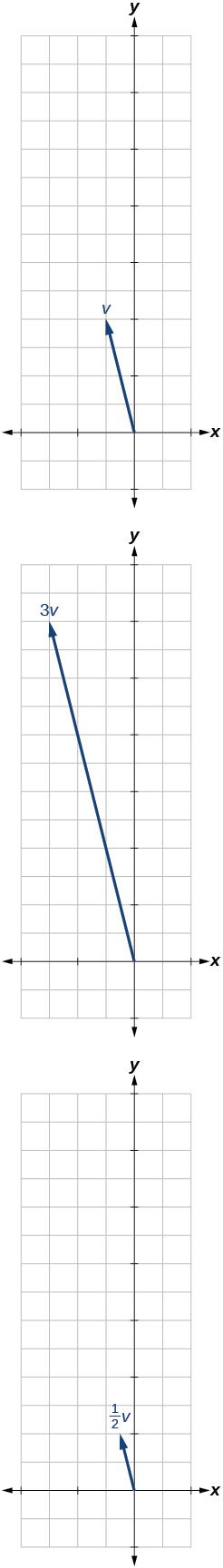$⟨-3,-2⟩$

For the following exercises, use the vectors shown to sketch u + v , u v , and 2 u .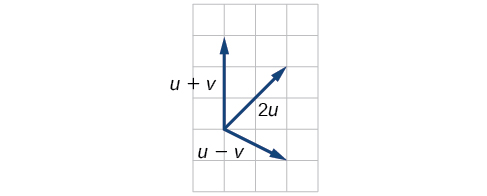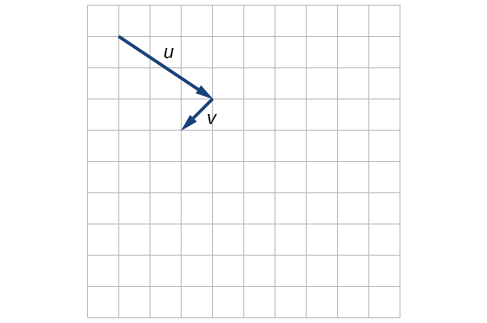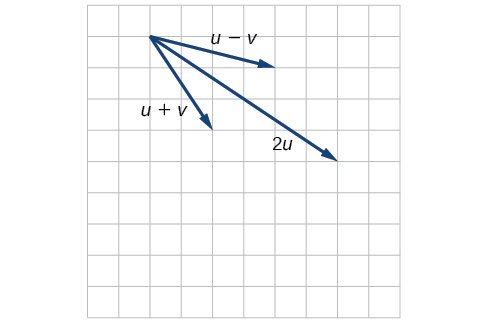For the following exercises, use the vectors shown to sketch 2 u + v .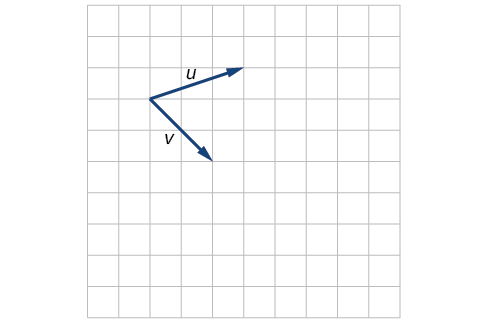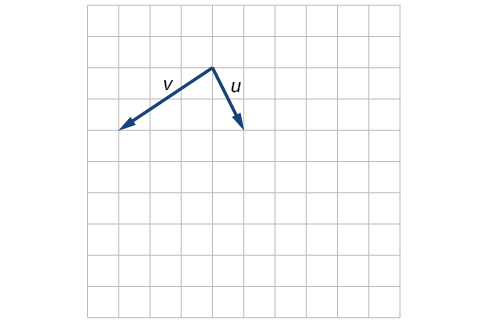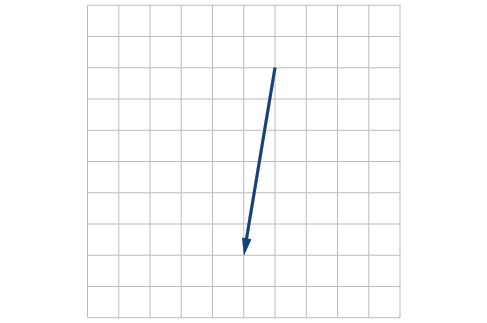For the following exercises, use the vectors shown to sketch u − 3 v .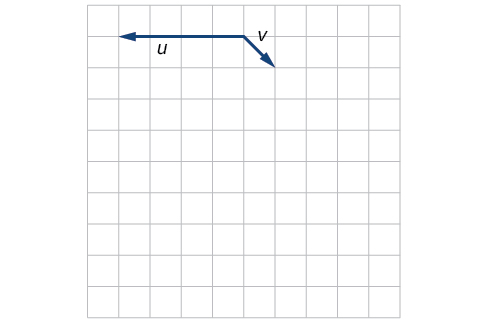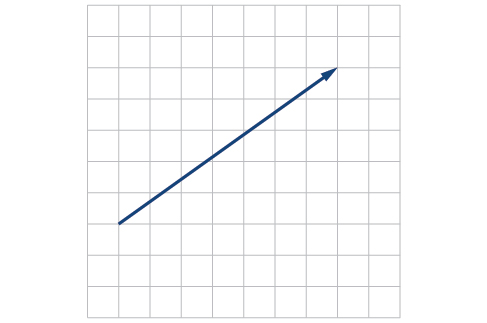For the following exercises, write the vector shown in component form.

bsc F. y algebra and trigonometry pepper 2
given that x= 3/5 find sin 3x
4
DB
remove any signs and collect terms of -2(8a-3b-c)
-16a+6b+2c
Will
Joeval
(x2-2x+8)-4(x2-3x+5)
sorry
Miranda
x²-2x+9-4x²+12x-20 -3x²+10x+11
Miranda
x²-2x+9-4x²+12x-20 -3x²+10x+11
Miranda
(X2-2X+8)-4(X2-3X+5)=0 ?
master
The anwser is imaginary number if you want to know The anwser of the expression you must arrange The expression and use quadratic formula To find the answer
master
The anwser is imaginary number if you want to know The anwser of the expression you must arrange The expression and use quadratic formula To find the answer
master
Y
master
master
Soo sorry (5±Root11* i)/3
master
Mukhtar
explain and give four example of hyperbolic function
What is the correct rational algebraic expression of the given "a fraction whose denominator is 10 more than the numerator y?
y/y+10
Mr
Find nth derivative of eax sin (bx + c).
Find area common to the parabola y2 = 4ax and x2 = 4ay.
Anurag
A rectangular garden is 25ft wide. if its area is 1125ft, what is the length of the garden
to find the length I divide the area by the wide wich means 1125ft/25ft=45
Miranda
thanks
Jhovie
What do you call a relation where each element in the domain is related to only one value in the range by some rules?
A banana.
Yaona
given 4cot thither +3=0and 0°<thither <180° use a sketch to determine the value of the following a)cos thither
what are you up to?
nothing up todat yet
Miranda
hi
jai
hello
jai
Miranda Drice
jai
aap konsi country se ho
jai
which language is that
Miranda
I am living in india
jai
good
Miranda
what is the formula for calculating algebraic
I think the formula for calculating algebraic is the statement of the equality of two expression stimulate by a set of addition, multiplication, soustraction, division, raising to a power and extraction of Root. U believe by having those in the equation you will be in measure to calculate it
Miranda
state and prove Cayley hamilton therom
hello
Propessor
hi
Miranda
the Cayley hamilton Theorem state if A is a square matrix and if f(x) is its characterics polynomial then f(x)=0 in another ways evey square matrix is a root of its chatacteristics polynomial.
Miranda
hi
jai
hi Miranda
jai
thanks
Propessor
welcome
jai
What is algebra
algebra is a branch of the mathematics to calculate expressions follow.
Miranda
Miranda Drice would you mind teaching me mathematics? I think you are really good at math. I'm not good at it. In fact I hate it. 😅😅😅
Jeffrey
lolll who told you I'm good at it
Miranda
something seems to wispher me to my ear that u are good at it. lol
Jeffrey
lolllll if you say so
Miranda
but seriously, Im really bad at math. And I hate it. But you see, I downloaded this app two months ago hoping to master it.
Jeffrey
which grade are you in though
Miranda
oh woww I understand
Miranda
Jeffrey
Jeffrey
Miranda
how come you finished in college and you don't like math though
Miranda
gotta practice, holmie
Steve
if you never use it you won't be able to appreciate it
Steve
I don't know why. But Im trying to like it.
Jeffrey
yes steve. you're right
Jeffrey
so you better
Miranda
what is the solution of the given equation?
which equation
Miranda
I dont know. lol
Jeffrey
Miranda
JeffreyByByBy Nick SwainBy OpenStaxBy JavaChamp TeamBy OpenStaxBy Madison ChristianBy Steve GibbsBy Rylee MinllicBy Samuel MaddenBy David GeltnerBy Rhodes